## Sunday, November 17, 2013

### HP Prime Programming Tutorial #5: STARTAPP, STARTVIEW, RGB

Today's session is about starting other apps in a program and using colors.

Defining equations in the Program Editor and Home

The equation must be a string and be stored to the appropriate designated variable.

F# is for functions of X. (Function app).
R# is for polar functions of θ. (Polar app).
U# is for sequences of N, N-1, N-2. (Sequence app).
X# and Y# for parametric equations of T. (Parametric App)
V# for open statements and equations in the Advanced Graphing App, which The independent variables are X and Y.

# is a digit 0-9.

Defining equations this way leaves them uncheck. If you want them plotted or accessed in Num View, you will need to check them.

Example:
F1:="2*X^3" stores the function f(x) = 2*x^3 in Function 1.

R5:="A*SIN(θ)" stores the polar function r(θ) = A*sin(θ) in Polar Function 5, with A being what value stored in it.

STARTAPP

STARTAPP(application name in quotes);

Starts the named App. The calculator points the screen to the default view (Plot, Symb, Num).

Access: Cmds, 4. App Functions, 2. STARTAPP

CHECK and UNCHECK

Checks and unchecks specific equation or function (0-9) in the current app. For example, if you are in the Function app, CHECK(1) activates F. As you should expect, UNCHECK(1) turns F1 off.

What does CHECK and UNCHECK affect?
1. Whether a function is plotted in Plot view.
2. Whether a function is analyzed in Num view.

Access for CHECK: Cmds, 4. App Functions, 1. CHECK
Access for UNCHECK: Cmds, 4. App Functions, 4. UNCHECK

STARTVIEW

Instructs the HP Prime to go to a certain view. It has two arguments, the view number and a redraw number.

Common view numbers include (not all inclusive):
-2 = Modes screen
-1 = Home
0 = Symbolic (Symb)
1 = Plot
2 = Numeric (Num)
3 = Symbolic Setup
4 = Plot Setup
5 = Numeric Setup
6 = App Information
7 = The Views Key
8 = first special view
9 = second special view
Etc..

The redraw number is either 0 or non-zero. 0 does not redraw the screen, anything else does. I recommend the latter.

Syntax: STARTVIEW(view number, redraw number)

Access: Cmds, 4. App Functions, 3. STARTVIEW

RGB

Returns an integer code pertaining to a color's RGB code. This is super useful for drawing and text writing.

Syntax: RGB(red, green, blue, alpha)

Red: Intensity of Red, 0-255
Green: Intensity of Green, 0-255
Blue: Intensity of Blue, 0-255
Alpha: (optional) Opacity (up to 128).

RGB codes:
Blue: RGB(0,0,255)
Violet: RGB(143,255,0)
Dark Green: RGB(0,128,0)
Orange: RGB(255,127,0)
Yellow: RGB(0,255,255)
Red: RGB(255,0,0)
White: RGB(255,255,255)
Black: RGB(0,0,0)
Gray: RGB(129,128,128)
Brown: RGB(150,75,0)
Light Blue: RGB(173,216,330)

For other colors, RGB can be found on various sites on the Internet, including Wikipedia.

Access: Cmds, 2. Drawing, 5. RGB

Tip: Change a color of a graph

Use the syntax

F#(COLOR):=RGB(red,blue,green,[alpha]);

F stands for the designated function type (F for function, R for polar, etc)
# is the digit 0-9.

Example:
F8(COLOR):=RGB(0,0,255)
makes the function F8 plot in blue.

This is a lot, but this is doable. Let's see all these commands and tips in action and create some magic.

Conic Drawing for HP Prime

Draws the conic section for the general equation

Ax^2 + By^2 + Cxy + Dx + Ey + F = 0

You can choose the color how the conic section is plotted, from red, blue, orange, and green. (Game show enthusiasts take note of the order of the colors I listed... ;) ).

EXPORT CONIC()
BEGIN
LOCAL cr, cg, cb, I;
INPUT({A,B,C,D,E,F},
"Ax^2+By^2+Cxy+Dx+Ey+F", { }, { },
{0,0,0,0,0,0});

// Colors
CHOOSE(I, "Choose a Color",
"Red","Blue","Orange","Green");
cr:={255,0,255,0};
cg:={0,0,127,255};
cb:={0,255,0,0};

V1:="A*X^2+B*Y^2+C*X*Y+D*X+E*Y+F=0";
V1(COLOR):=RGB(cr(I),cg(I),cb(I));
CHECK(1);
// Plot View
STARTVIEW(1,1);
END;

Below are some examples. Remember the form:

Ax^2 + By^2 + Cxy + Dx + Ey + F = 0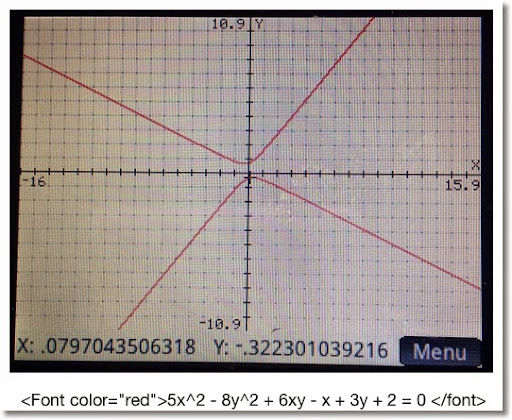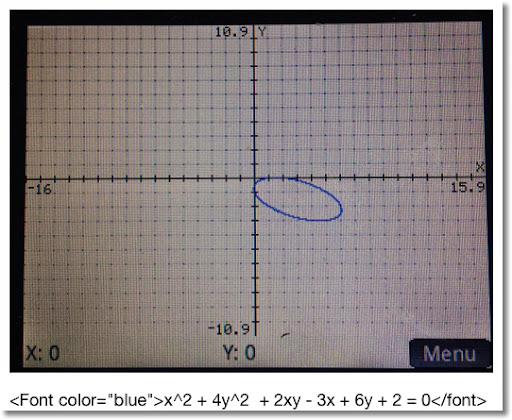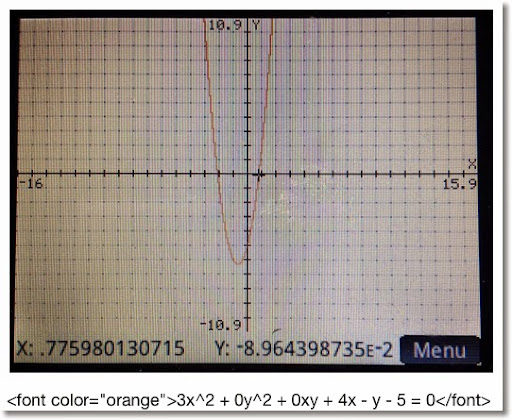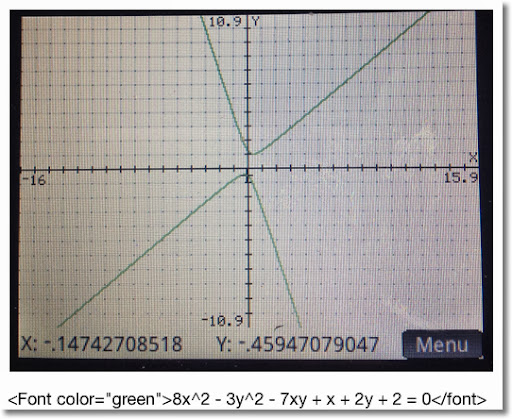Projectile Motion for HP Prime

This program calculates range and height of a projectile, and plots its path. The program sets the mode into Degrees (HAngle=1) and the calculator to the Parametric app.

Equations:
x = V * cos θ * t
y = V * sin θ * t - .5 * g * t^2

Where
V = initial velocity
θ = initial degree of flight
g = Earth gravitation constant (9.80665 m/s^2, ≈32.17404 ft/s^2)

Air resistance is not factored, so we are dealing with ideal conditions. How much the projectile represents reality varies, where factors include the object being projected, the temperate and pressure of the air, and the weather.

EXPORT PROJ13()
BEGIN
LOCAL M, str;
// V, G, θ are global
// Degrees
HAngle:=1;

CHOOSE(M, "Units", "SI", "US");
IF M==1 THEN
str:="m";
G:=9.80665;
ELSE
str:="ft";
G:=32.17404;
END;

INPUT({V, θ}, "Data",
{"V:","θ:"},
{"Initial Velocity in "+str+"/s",
"Initial Angle in Degrees"});

X1:="V*COS(θ)*T";
Y1:="V*SIN(θ)*T-.5*G*T^2";

STARTAPP("Parametric");
CHECK(1);
Xmin:=0
// Range
Xmax:=V^2/G*SIN(2*θ);
Ymin:=0
// Height
Ymax:=(V^2*SIN(θ)^2)/(2*G);
MSGBOX("Range: "+Xmax+" "+str+", "
+", Height: "+Ymax+" "+str);
// Plot View
STARTVIEW(1,1);
END;

Below are screen shots from an example with V = 35.25 m/s and θ = 48.7°.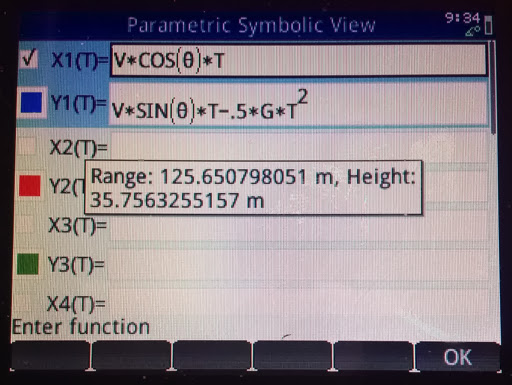This concludes this session of the tutorials. Shortly I will have Part 6 up, which has to do routines.

I am catch-up mode, still. But then again I always feel like there is too much to do and too little time. LOL

See you soon!

Eddie

This blog is property of Edward Shore. 2013

1.Hey, is there a way to star the Geometry app? Im trying to plot some points, that are obtained after solving a cZeros inside a for statement. What Im trying is to vary a coeficient of a poly and then plot its complex roots i a 2D-Diagram. In the geometry app semms to be possible, but I cant find how to program anything to be plotted there. Any ideas?

1.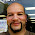I want to look into this further. I am thinking that we would use both the STARTAPP("Geometry") and point(x,y) commands.

Eddie

2.Pedro,

I haven't been able to use the Geometry app, but here is an idea on how to approach plotting the points of the roots:

EXPORT TEST1061(A,B,C)
BEGIN
LOCAL a,b,c,d,lst,l1,l2;
// Solve
lst:=CAS.cZeros(A*X^2+B*X+C,X);
// Extraction
a:=RE(lst(1));
b:=IM(lst(1));
c:=RE(lst(2));
d:=IM(lst(2));
// Plot setup
RECT();
Xmin:=MIN(a,c)-1;
Xmax:=MAX(a,c)+1;
Ymin:=MIN(b,d)-1;
Ymax:=MAX(b,d)+1;
// Plot
LINE(0,Ymin,0,Ymax,#202020h);
LINE(Xmin,0,Xmax,0,#202020h);
// Make the points stand out
l1:=C→PX(a,b);
RECT_P(l1(1)-5,l1(2)-5,l1(1)+5,
l1(2)+5,#80,#FF);
l2:=C→PX(c,d);
RECT_P(l2(1)-5,l2(2)-5,l2(1)+5,
l2(2)+5,#80,#FF);
WAIT(0);
RETURN lst;
END;

2.Thanks! I have not yep been able to start the geometry app, but managed to make a little program that will give me a list of values for the path of each root while I change a value of gain K. Then I copy each list and plot separated with the plotlist function in the geometry app. So here is my code for plotting the Root Locus in the HP Prime:

(A,B)->BEGIN
LOCAL I,L,J,G,K,N,TF,TFI,TFF,TF1;
purge(L1,L2,L3,L4,L5);
TFF:=cZeros(B);
TFI:=mat2list(TFF);
TF:=EDITLIST(TFI);
PRINT(TF);
N:=SIZE(TF);
PRINT(N);
G:=1;
FOR K FROM 1 TO 50 STEP 0.1 DO
TF1:=cZeros(B+K*A);
PRINT(TF1);
IF N = 1 THEN L1(G):=[RE(TF1(1)),IM(TF1(1))]; END ;
IF N = 2 THEN
L1(G):=[RE(TF1(1)),IM(TF1(1))];
L2(G):=[RE(TF1(2)),IM(TF1(2))] END ;
IF N = 3 THEN
L1(G):=[RE(TF1(1)),IM(TF1(1))];
L2(G):=[RE(TF1(2)),IM(TF1(2))];
L3(G):=[RE(TF1(3)),IM(TF1(3))] END ;
IF N = 4 THEN
L1(G):=[RE(TF1(1)),IM(TF1(1))];
L2(G):=[RE(TF1(2)),IM(TF1(2))];
L3(G):=[RE(TF1(3)),IM(TF1(3))];
L4(G):=[RE(TF1(4)),IM(TF1(4))] END ;
IF N = 5 THEN
L1(G):=[RE(TF1(1)),IM(TF1(1))];
L2(G):=[RE(TF1(2)),IM(TF1(2))];
L3(G):=[RE(TF1(3)),IM(TF1(3))];
L4(G):=[RE(TF1(4)),IM(TF1(4))];
L5(G):=[RE(TF1(5)),IM(TF1(5))] END ;
G:=G+1;
END;;
PRINT();
RETURN([[L1],[L2],[L3],[L4],[L5]]);
END;

1.PS: That is a CAS program.
AND

That Editlist I put there so you can correct the cZeros function, because it sometimes do not return roots with multiplicity. Like when I use cZeros(x^2*(x-1)) it returns only (-1,0), instead of (-1,0,0).

If you find a way to plot it in the geometry app, let me know!
Cheers

2.Thanks, Pedro!

Eddie

3.Pedro,

Question: What are the inputs (A and B)? Thanks,

Eddie

4.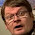// A MORE COMPACT VERSION
(A,B)->BEGIN
LOCAL I,L,J,G,K,N,TF,TFI,TFF,TF1;
purge(L1,L2,L3,L4,L5);
TFF:=cZeros(B);
TFI:=mat2list(TFF);
TF:=EDITLIST(TFI);
PRINT(TF);
N:=SIZE(TF);
PRINT(N);
G:=1;
FOR K FROM 1 TO 50 STEP 0.1 DO
TF1:=cZeros(B+K*A);
PRINT(TF1);
L1(G):=[RE(TF1(1)),IM(TF1(1))];
IF N > 1 THEN
L2(G):=[RE(TF1(2)),IM(TF1(2))] END ;
IF N > 2 THEN
L3(G):=[RE(TF1(3)),IM(TF1(3))] END ;
IF N > 3 THEN
L4(G):=[RE(TF1(4)),IM(TF1(4))] END ;
IF N > 4 THEN
L5(G):=[RE(TF1(5)),IM(TF1(5))] END ;
G:=G+1;
END;;
PRINT();
RETURN([[L1],[L2],[L3],[L4],[L5]]);
END;

3.Sorry. A and B would be the A= numerator and B denominator of the closed loop transfer function that you wish to plot. It comes from the complete equation:
1+K.A/B=0, or where G(s)=A/B, this equation is the transfer function of a generic closed loop system in function of a gain K. See more in:
http://en.m.wikipedia.org/wiki/Root_locus
This is part of the control systems theory. Its one of the subjects im taking this semester. :)
If I can find a way to plot it like the graphic example in the link above, would be incredible. Greetings

1.A and B must also be a poly using x. Ex: x-1 as A and x^2+2x+5 as B input. Note the use of lower case x.

4.Try the Parametric instead of the Geometry App. I used my HP48GX's Parametric Plot when I implemented a Root Locus program 20 years ago.

5.Hi!
I'm not managed this app.Can someone help me? Thanks

6.Hi!
I'm not managed this app.Can someone help me? Thanks

7.Hi!, Pedro Souza:
Can you please, write your program, complete, for Check Ok.
Thank you, in advance.
Maké

8.I think this is one of the most significant information for me.And i’m glad reading your article.But should remark on some general things, the web site style is perfect,the articles is really great : D. Good job…
IWIN89

### Retro Review: Radio Shack EC-2024

Retro Review:  Radio Shack EC-2024 Quick Facts Company:  Radio Shack Years in Production: Around 1994 Original Price: \$24.99 Bat...